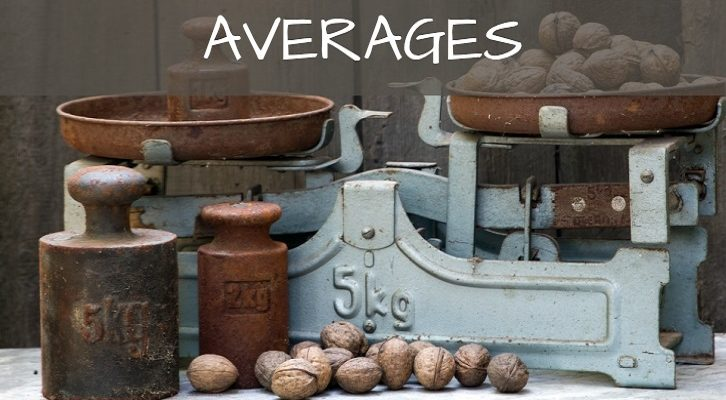# CAT Averages

Question
1.       5. Scores in a classroom are broken into 5 different ranges, 51-60, 61-70, 71-80, 81-90 and 91-100. The number of students who have scored in each range is given below
51 -60 – 3 students
61 -70 – 8 students
71 -80 – 7 students
81 -90 – 4 students
91 -100 – 3 students
Furthermore, we know that at least as many students scored 76 or more as those who scored below 75. What is the minimum possible average overall of this class?
1. 72
2. 71.2
3. 70.6
4. 69.2
Let’s employ the idea of a total of 25 students (all of the same weight) sitting on a see-saw, which has numbers from 51 to 100 marked on it. At least as many students are sitting on 76 (or to its right), as there are sitting to the left of 75. Now this means that you can have only one person sitting to the left of 75 and all the rest sitting beyond 76. But you can’t do that, as you have other constraints as well.
First of all, you have to seat 3 students from 51 to 60, and 8 students from 61 to 70. Secondly, you also have to make sure that the average is the least. This means that the see-saw should be tilting as much to the left as possible, which in turn means that the number of people sitting to the left of 75 should be the highest possible.
This makes it 12 students to the left of 75, and the remaining 13 students on 76 or to its right.
Next, how do you ensure that the average is least, i.e. how do you ensure that the balance tilts as much as possible to the left? Make each student score as little as possible given the constraints.
So, the first 3 students only score 51 each. The next 8 students score only 61 each. 11 Students are now fixed. The 12th student has to be below 75, so seat him on 71. The remaining 6 students (who are in the 71 to 80 range) have to score 76. The next 4 score 81 and the next 3 score 91.
This would give you the least average.
The lowest possible average would be:
= [(3 * 51) + (8 * 61) + 71 + (6 * 76) + (4* 81) +  (3 * 91)]/25
= 70.6
1.Karthikeyan Chockalingam says: